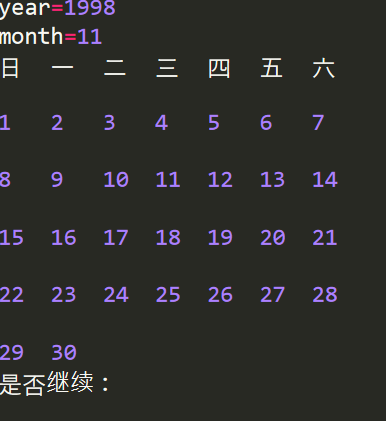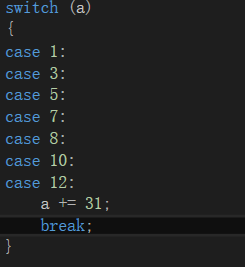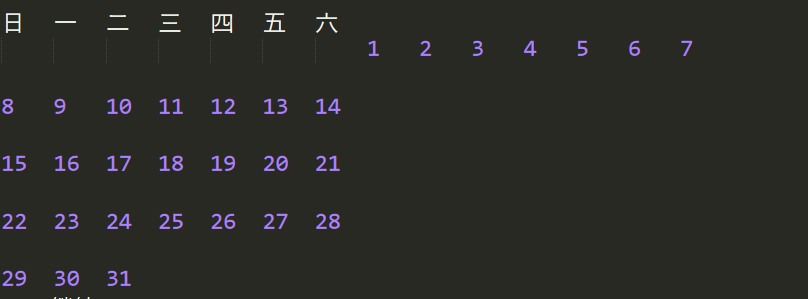# Python實戰練習——列印日曆教程

Python實戰教程，用Python做列印日曆的小程式，使用者可以通過輸入年月信息，程式將會輸出這個月的日曆 ...1.isleapyear（）

（year%4==0 and year%100！=0）or year%400==0

```def IsleapYear(year):
flag =False
if(year%4==0 and year%100!=0) or year%400==0:
flag = True
return flag```

2.calculation（）

`if isleapyear（s_year）：`

c語言中的分支結構有 if 與 switch兩種

`if（month==1||month==3||month==5||month==7||month==8||month==10||month==12）`在python中則可以通過組合數據類型之集合的方式來簡化

```Lunar=(1,3,5,7,8,10,12)
if s_month in Lunar:
sum += 31
elif s_month==2:
if IsleapYear(year):
sum+=29
else:
sum+=28
else:
sum+=30```

```def calculation(year,month):
sum = 0
s_year=1990
while s_year < year-1:
s_year +=1
if IsleapYear(s_year):
sum+=366
else:
sum+=365

s_month=1
while s_month<month:
if s_month in Lunar:
sum += 31
elif s_month==2:
if IsleapYear(year):
sum+=29
else:
sum+=28
else:
sum+=30
s_month+=1

return sum```

3.display（）

```def display(sum,year,month):
#第一步
week=(sum+1)%7
#第二步
print("日\t一\t二\t三\t四\t五\t六")
#第三步
count = 0
space = 0
while space<=week:
space+=1
count+=1
print("\t",end="")
if count%7==0:
print("\n",end="")
#第四步
if month in Lunar:
day=31
elif month==2:
if IsleapYear(year):
day=29
else:
day=28
else:
day=30
days=1
while days<=day:
print(days,"\t",end="")
days+=1
count+=1
if count %7 ==0:
print("\n")    ```4.main（）

main函數需要設置可以接受年月信息，同時傳參調用剛剛的函數

```def main():
year =int( input("year="))
if year<1990 or year>9999:
print("year輸入錯誤")
main()
month = int(input("month="))
if month<1 or month > 12:
print("month輸入錯誤")
main()
sums=calculation(year,month)
display(sums,year,month)

if __name__=="__main__":
while True:
main()
choose=input("\n是否繼續：")
if choose in("n","N"):
break```

```Lunar=(1,3,5,7,8,10,12)

def IsleapYear(year):
flag =False
if(year%4==0 and year%100!=0) or year%400==0:
flag = True
return flag

def calculation(year,month):
sum = 0
s_year=1990
while s_year < year-1:
s_year +=1
if IsleapYear(s_year):
sum+=366
else:
sum+=365

s_month=1
while s_month<month:
if s_month in Lunar:
sum += 31
elif s_month==2:
if IsleapYear(year):
sum+=29
else:
sum+=28
else:
sum+=30
s_month+=1

return sum

def display(sum,year,month):
week=(sum+1)%7
if month in Lunar:
day=31
elif month==2:
if IsleapYear(year):
day=29
else:
day=28
else:
day=30

print("日\t一\t二\t三\t四\t五\t六")
count = 0
space = 0
while space<=week:
space+=1
count+=1
print("\t",end="")
if count%7==0:
print("\n",end="")
days=1
while days<=day:
print(days,"\t",end="")
days+=1
count+=1
if count %7 ==0:
print("\n")
def main():
year =int( input("year="))
if year<1990 or year>9999:
print("year輸入錯誤")
main()
month = int(input("month="))
if month<1 or month > 12:
print("month輸入錯誤")
main()
sums=calculation(year,month)
display(sums,year,month)

if __name__=="__main__":
while True:
main()
choose=input("\n是否繼續：")
if choose in("n","N"):
break```• 智力題目有三個容積分別為3升、5升、8升的水桶，其中容積為8升的水桶中裝滿了水，容積為3升和容積為5升的水桶都是空的。三個水桶都沒有刻度，現在需要將大水桶中的8升水等分成兩份，每份都是4升水，附加條件是只能這三個水桶，不能藉助其他輔助容器。“恩，是的，這是一個很經典的問題。”“然而，我們並不能想全， ...
• 今天，在Anaconda prompt啟動python遇到瞭如下錯誤： UnicodeDecodeError: ‘gbk’ codec can’t decode byte 0xaf in position 553: illegal multibyte sequence 看了看出錯跟蹤，查看瞭如下位置 ...
• 許多小伙伴對於java中的三種初始化塊的執行順序一直感到頭疼，接下來我們就來分析一下這三種初始化塊到底是怎麼運行的。有些公司也會將這個問題作為筆試題目。 下麵通過一段代碼來看看創建對象時這麼初始化塊是如何運行的 package com.hxy; public class CodeBlock{ pub ...
• 字元串或串(String)是由數字、字母、下劃線組成的一串字元。一般記為 s=“a1a2···an”(n>=0)。它是編程語言中表示文本的數據類型。在程式設計中，字元串（string）為符號或數值的一個連續序列，如符號串（一串字元）或二進位數字串（一串二進位數字）。 String類型你一定不陌生，畢 ...
• 我們知道，swoole中有兩大進程，分別是 master 主進程和 manager 管理進程。 其中 master 主進程中會有一個主 reactor 線程和多個 reactor 線程，主要的作用就是用來維護TCP連接，處理網路IO，收發數據。 而 manager 管理進程，作用則是 fork 和管 ...
• 1. random模塊 導入的是random模塊，格式是： import random 1.1 隨機小數 取隨機小數 : 數學計算。 print(random.random()) # 取0-1之間的小數print(random.uniform(1,2)) # 取1-2之間的小數 1.2 隨機整數 取 ...
• 一、阻塞隊列：用於保存等待執行的任務。在阻塞隊列中，線程阻塞的兩種情況： 1.當隊列中沒有數據的情況下，消費者端的所有線程都會被自動阻塞（掛起），直到有數據放入隊列。 2.當隊列中填滿數據的情況下， 生產者端的所有線程都會被自動阻塞，知道隊列中有空位置，線程被自動喚醒。 二、阻塞隊列的主要方法 拋出 ...
• 一、實習內容 選擇一個調度演算法，實現處理器調度。 二、實習目的 在採用多道程式設計的系統中，往往有若幹個進程同時處於就緒狀態。當就緒進程個數大於處理器數時，就必須依照某種策略來決定哪些進程優先占用處理器。本實習模擬在單處理器情況下的處理器調度，幫助學生加深瞭解處理器調度的工作。 三、實習題目 本實習 ...

• C 語法糖——持續更新 1. return的switch寫法 ...
• 0. 前言 繼上一篇，以及上上篇，我們對SqlSugar有了一個大概的認識，但是這並不完美，因為那些都是理論知識，無法描述我們工程開發中實際情況。而這一篇，將帶領小伙伴們一起試著寫一個能在工程中使用的模板類。 1. 創建一個Client SqlSugar在操作的時候需要一個Client，用來管理數據 ...
• 1 class Program 2 { 3 static void Main(string[] args) 4 { 5 //數組：長度不可變，類型單一 6 //ArrayList集合：長度可以任意改變，類型可以不單一 7 8 //創建一個ArrayList對象 9 ArrayList mylist ...
• .NET 程式下銳浪報表 (Grid++ Report) 的綠色發佈指南 在銳浪報表官方為 CSharp 編寫的開發文檔：“在C#與VB.NET中開始使用說明.txt” 中，關於發佈項目是這麼描述的： ★發佈你的項目，用VS.NET製作安裝程式：1、先創建安裝項目：在解決方案資源管理器的根節點上點右 ...
• 執行代碼清理時，可以點擊那個掃把小圖片，會按照預設的第一種配置文件來自動修複。也可以點擊下拉三角符合，選擇不同的配置文件，然後進行修複。或者快捷鍵Ctrl+K，Ctrl+E。 針對每一項配置的說明： 刪除不必要的using 儘可能將私有欄位設置為只讀 刪除不必要的類型轉換（針對強類型轉換），像Con ...
• 1.概念簡述 （1）AR模型 AR 模型(auto regressive model)自回歸模型，模型參量法高解析度譜分析方法之一，也是現代譜估計中常用的模型。 用AR模型法求信具體作法是： ①選擇AR模型，在輸入是衝激函數或白雜訊的情況下，使其輸出等於所研究的信號，至少，應是對該信號的一個好的近似 ...
• 4.元組 元組的主要特性為： 1.元組在創建之後，具有不可以更改的特性，因此不能直接給元組的元素賦值 2.元組的元素類型可以為任意類型，如字典、字元串、列表等 3.元組常用於在程式的整個生命周期中都不變的場景中 4.1 常用方法 元組大小和內容在定義賦值之後，就不可更改，常用的方法如下所示： cou ...
• 老孟導讀：今天分享一個類似“孔雀開屏”的動畫效果，打開新的頁面時，新的頁面從屏幕右上角以圓形逐漸打開到全屏。 先來看下具體的效果 不知道這種效果大家叫什麼名字？如果有更合適的名字可以在評論處告訴我，下麵來說下如何實現此效果。 在使用Navigator進入一個新的頁面時，通常用法如下： 就包含了切換頁 ...
• hashCode() 和equals() 方法的重要性體現在什麼地方？ Java中的HashMap使用hashCode()和equals()方法設置值，根據鍵獲取值的時候也會用到這兩個方法。 怎樣 設置 的值？ hashCode()獲得 hash值。而hash值用來確定hashmap中內部 Node ...
• IDEA一些不錯的插件分享 目錄 IDEA一些不錯的插件分享 插件集合 CamelCase Translation LiveEdit MarkDown Navigator Jrebel CheckStyle IDEA Alibaba Java Coding Guidelines Ideavim Ma ...